## Example Questions

### Example Question #1 : Trigonometry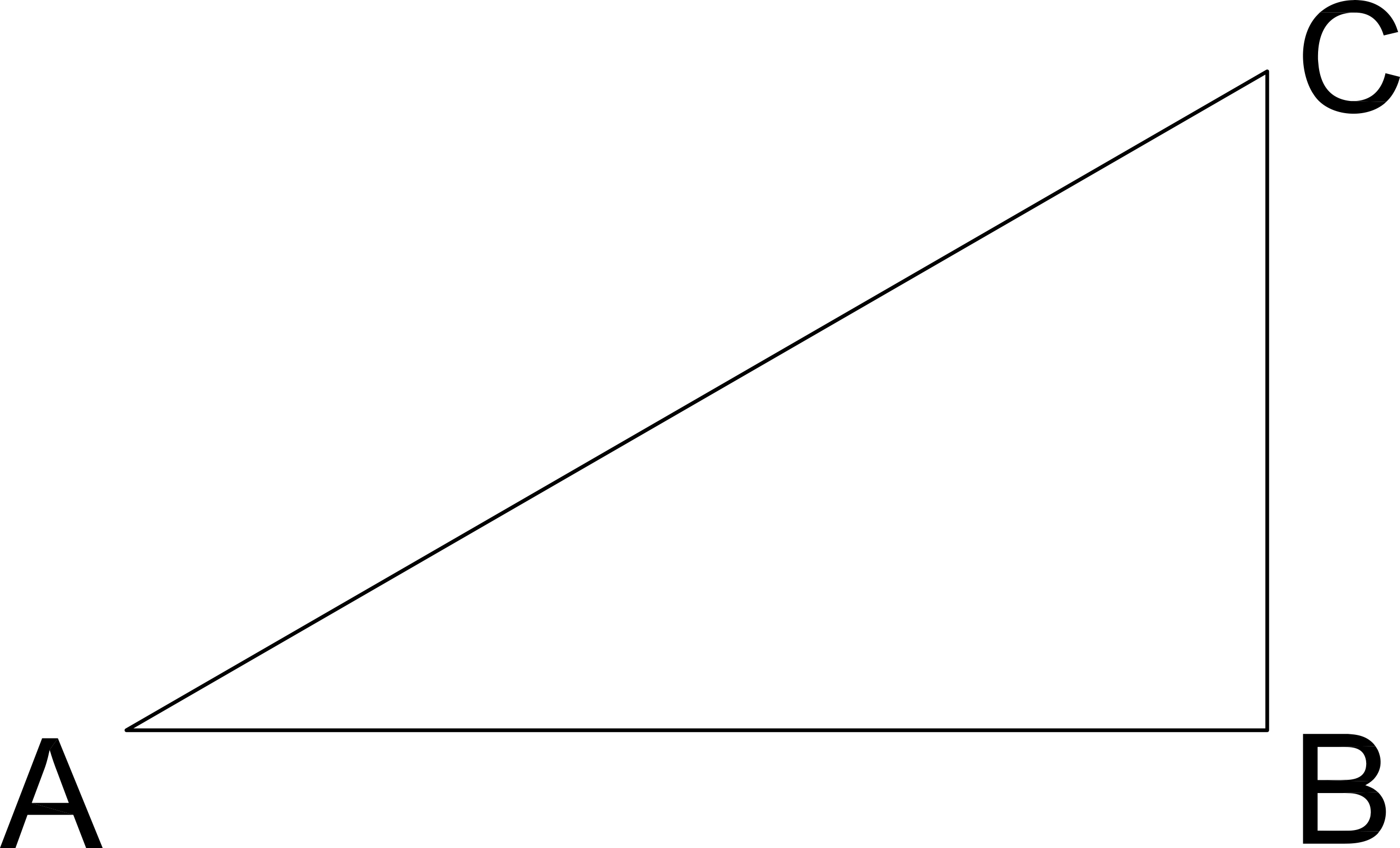What is the tangent of C in the given right triangle??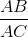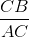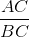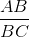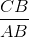Explanation:

### Example Question #2 : Trigonometry

Consider a right triangle with an inner angle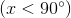.

If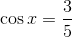and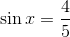what is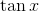?Explanation:

The tangent of an angle x is defined as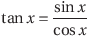Substituting the given values for cos x and sin x, we get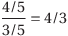### Example Question #1 : TrigonometryTriangle ABC shown is a right triangle. If the tangent of angle C is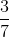, what is the length of segment BC?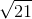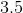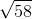Explanation:

Use the definition of the tangent and plug in the values given:

tangent C = Opposite / Adjacent = AB / BC = 3 / 7

Therefore, BC = 7.

### Example Question #4 : Trigonometry

If the sine of an angle equals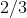, and the cosine of the same angle equals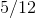, what is the tangent of the angle?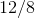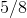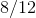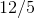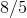Explanation: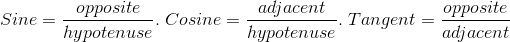The cosine of the angle isand since that is a reduced fraction, we know the hypotenuse isand the adjacent side equals.

The sine of the angle equals, and since the hyptenuse is alreadywe know that we must multiply the numerator and denominator byto get the common denominator of.  Therefore, the opposite side equals.

Since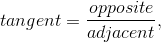, the answer is